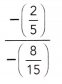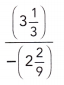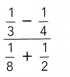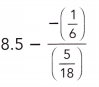# Math in Focus Grade 7 Chapter 2 Review Test Answer Key

Go through the Math in Focus Grade 7 Workbook Answer Key Chapter 2 Review Test to finish your assignments.

## Math in Focus Grade 7 Course 2 A Chapter 2 Review Test Answer Key

Concepts and Skills

Evaluate each sum or difference.

Question 1.
-6 + 14
If the signs are different we have to subtract the numbers.
14 – 6 = 8
Thus -6 + 14 = 8

Question 2.
-25 + (-9)
If the signs are same then you have to add the numbers.
-25 – 9 = -34

Question 3.
52 + (-52)
52 – 52 = 0

Question 4.
46 + (-17) + 38
46 + 38 – 17
84 – 17 = 67

Question 5.
-80 + 63 + (-24)
First, arrange all the negative signs in one place
-80 – 24 + 63
Here greatest value has a negative sign so the results will be negative.
-124 + 63 = -61

Question 6.
35 – 140
Given,
35 – 140
140 – 35 = 105
Here greatest value has a negative sign so the results will be negative i.e., -105.

Question 7.
-61 – 28
Given,
-61 – 28
If the signs are the same then you have to add the numbers.
61 + 28 = 89
-61 – 28 = -89

Question 8.
128 – (-73)
Given,
128 – (-73)
128 + 73 = 201

Question 9.
-8 – (-50)
Given,
-8 – (-50)
-8 + 50
50 – 8 = 42

Question 10.
$$\frac{1}{4}$$ – $$\frac{7}{8}$$
Given,
$$\frac{1}{4}$$ – $$\frac{7}{8}$$
Here the denominators of both the fractions are not same.
The LCM of 4 and 8 is 8.
$$\frac{2}{8}$$ – $$\frac{7}{8}$$
(2-7)/8 = – $$\frac{5}{8}$$

Question 11.
14$$\frac{2}{3}$$ – 8$$\frac{4}{5}$$
Given,
14$$\frac{2}{3}$$ – 8$$\frac{4}{5}$$
14 – 8 = 6
Now subtract the fractions
$$\frac{2}{3}$$ – $$\frac{4}{5}$$
Here the denominators of both the fractions are not same.
LCM of 3 and 5 is 15.
$$\frac{10}{15}$$ – $$\frac{12}{15}$$ = – $$\frac{2}{15}$$

Question 12.
-6$$\frac{1}{7}$$ + 3$$\frac{5}{14}$$
Given,
-6$$\frac{1}{7}$$ + 3$$\frac{5}{14}$$
– 6 + 3 = -3
$$\frac{5}{14}$$ – $$\frac{1}{7}$$
Here the denominators of both the fractions are not same.
LCM of 14 and 7 is 14.
$$\frac{5}{14}$$ – $$\frac{2}{14}$$ = $$\frac{3}{14}$$
-3 + $$\frac{3}{14}$$ = -2$$\frac{11}{14}$$

Evaluate each product or quotient.

Question 13.
-5 • 11
Given,
-5 • 11
minus × plus = minus
So, the result will be negative
-5 × 11 = -55

Question 14.
-30 • (-4)
Given,
-30 • (-4)
minus × minus = plus
30 × 4 = 120

Question 15.
144 ÷ (-6)
Given,
144 ÷ (-6)
144 ÷ 6 = 24
Put negative sign to the obtained answer.
144 ÷ (-6) = -24

Question 16.
-48 ÷ (-3)
Given,
-48 ÷ (-3)
First cancel the negative signs
We get
48 ÷ 3 = 16
Thus -48 ÷ (-3) = 161

Question 17.
-126 ÷ 9
Given,
-126 ÷ 9
Remove the negative sign and perform division operation
126 ÷ 9 = 14
Put a negative sign to the obtained answer.
-126 ÷ 9 = -14

Question 18.
104 ÷ (-8)
Given,
104 ÷ (-8)
Remove the negative sign and perform division operation
104 ÷ 8 = 13
Put a negative sign to the obtained answer.
104 ÷ (-8) = -13

Question 19.
–$$\frac{5}{8}$$ • $$\frac{8}{15}$$
Given,
–$$\frac{5}{8}$$ • $$\frac{8}{15}$$
Cancel 8 and 8 we get 1 and cancel 5 by 15 we get 3.
So, the answer is –$$\frac{1}{3}$$

Question 20.
–$$\frac{3}{4}$$ • $$\left(-1 \frac{2}{3}\right)$$
Given,
–$$\frac{3}{4}$$ • 1 $$\frac{2}{3}$$
–$$\frac{3}{4}$$ • 1 $$\frac{2}{3}$$ = –$$\frac{3}{4}$$ × $$\frac{5}{3}$$
–$$\frac{3}{4}$$ × $$\frac{5}{3}$$ = $$\frac{15}{12}$$ = $$\frac{5}{4}$$
$$\frac{5}{4}$$ can be written as 1 $$\frac{1}{4}$$

Question 21.
5$$\frac{1}{4}$$ ÷ $$\left(-\frac{7}{12}\right)$$
Given,
5$$\frac{1}{4}$$ ÷ $$\left(-\frac{7}{12}\right)$$
$$\frac{21}{4}$$ ÷ –$$\frac{7}{12}$$
= (21 × 12)/(4 × 7)
= -252/28
= -9
5$$\frac{1}{4}$$ ÷ $$\left(-\frac{7}{12}\right)$$ = -9

Question 22.
-4$$\frac{4}{5}$$ ÷ 1$$\frac{1}{3}$$
Given,
-4$$\frac{4}{5}$$ ÷ 1$$\frac{1}{3}$$
$$\frac{24}{5}$$ ÷ $$\frac{4}{3}$$
= (24 × 3)/(5 × 4)
= 72/20
= 3 $$\frac{3}{5}$$

Question 23.Given,First cancel the negative signs
$$\frac{2}{5}$$ ÷ $$\frac{8}{15}$$
(2 × 15) ÷ (5 × 8)
30 ÷ 40
= 3/4

Question 24.Given,$$\frac{10}{3}$$ ÷ $$\frac{20}{9}$$
(10 × 9)/(3 × 20)
90/60 = 9/6 = 3/2
1 $$\frac{1}{2}$$

Evaluate each expression.

Question 25.
12.3 – 8.1 + 2$$\frac{1}{10}$$ • 0.4 – 1.6
Given,
12.3 – 8.1 + 2$$\frac{1}{10}$$ • 0.4 – 1.6
4.2 + $$\frac{21}{10}$$ × $$\frac{4}{10}$$ – 1.6
4.2 + $$\frac{82}{10}$$ – 1.6
4.2 + 8.2 – 1.6
12.4 – 1.6 = 10.8

Question 26.
(25.7 + 4) ÷ 3 + 0.82
Given,
(25.7 + 4) ÷ 3 + 0.82
29.4 ÷ 3 + 0.82
9.8 + 0.82 = 10.62

Question 27.
10 – 32.86 ÷ 5.3 + 7$$\left(\frac{81}{100}\right)$$
Given,
10 – 32.86 ÷ 5.3 + 7$$\left(\frac{81}{100}\right)$$
10 – 32.86 ÷ 5.3 + 7(0.81) = 9.47

Question 28.
3.25(0.9 – 0.74) + 6.3
Given,
3.25(0.9 – 0.74) + 6.3
3.25 (0.16) + 6.3
= 0.52 + 6.3
= 6.82

Question 29.Given,$$\frac{1}{3}$$ – $$\frac{1}{4}$$
LCM of 3 and 4 is 12
$$\frac{4}{12}$$ – $$\frac{3}{12}$$ = $$\frac{1}{12}$$
$$\frac{1}{8}$$ + $$\frac{1}{2}$$
LCM of 8 and 2 is 8
$$\frac{1}{8}$$ + $$\frac{4}{8}$$ = $$\frac{5}{8}$$
$$\frac{1}{12}$$ ÷ $$\frac{5}{8}$$
(1 × 8)/(12 × 5)
$$\frac{8}{60}$$
= $$\frac{2}{15}$$

Question 30.Given,–$$\frac{1}{6}$$ ÷ $$\frac{5}{18}$$
= –$$\frac{3}{5}$$
8.5 – (-$$\frac{3}{5}$$)
$$\frac{85}{10}$$ + $$\frac{3}{5}$$
LCM of 10 and 5 is 10.
$$\frac{85}{10}$$ + $$\frac{6}{10}$$ = $$\frac{91}{10}$$ = 9.1

Problem Solving

Question 31.
When you wake up on Monday, the temperature is -18°F. During the day, the temperature rises 13°F. By the time you wake up on Tuesday, it has dropped 19°F. What is the temperature when you wake up on Tuesday?

Question 32.
Tim dug a hole that was 16$$\frac{1}{2}$$ inches deep. He left a pile of dirt next to the hole that was 8$$\frac{3}{4}$$ inches high. Show how you could use subtraction to find the distance from the top of the pile of dirt to the bottom of the hole.
Given,
Length of the hole = 16$$\frac{1}{2}$$ inches
Length of the dirt = 8$$\frac{3}{4}$$ inches
The distance between the top of the pile to the bottom of the hole = ?
Since the dirt is beside the hole and not inside the hole, we can find it’s length using addition.
Distance from the top to the bottom = length of dirt + length of the hole
Length of dirt = 8×$$\frac{3}{4}$$ = 6 inches
Length of the hole = 16 × $$\frac{1}{2}$$ = 8 inches
Distance from the top of the dirt to the bottom of the hole = (6 + 8) inches
Distance from the top of the dirt to the bottom of the hole = 14 inches
Distance left = length of hole – length of dirt
Distance left = 8 – 6 = 2 inches

Question 33.
Daniel bought four bags of potatoes. The weight of the first bag was 2.6 pounds. The second bag weighed 0.4 pound less than twice the weight of the first bag. The third bag weighed 0.6 pound more than the first bag. The fourth bag weighed 0.3 pound less than the weight of the second bag. What is the average weight of the bags of potatoes that Daniel bought? Round your answer to the nearest tenth.
Given,
Weight of the first bag = 2.6 pounds
Weight of the second bag is 0.4 pounds less than twice the weight of the first bag.
W2 = 2W1 – 0.4 = 2(2.6 – 0.4) = 5.2 – 0.4 = 4.8 pounds
Weight of the third bag is 0.6 pounds more than that of the first bag.
W3 = W1 + 0.6 = 2.6 + 0.6 = 3.2 pounds
Weight of the fourth bag is 0.3 pounds less than that of the second bag.
W4 = W2 – 0.3 = 4.8 – 0.3 = 4.5 pounds
Therefore, the average weight of the bags of potatoes is given as the sum of all the weights and then divide the sum by 4.
Average weight = (W1 + W2 + W3 + W4)/4
= (2.6 + 4.8 + 3.2 + 4.5)/4
= 15.1/4
= 3.775

Question 34.
A hiker descended 320 feet in 40 minutes. Find the hiker’s average change in elevation per minute.
Given,
A hiker descended 320 feet in 40 minutes.
We have to find,
The hiker’s average change in elevation per minute.
According to the question.
We know that average speed is defined as distance divided by time.
Therefore,
Speed = Distance/Time
Here, the value of the distance is 320 feet and the value of time is 40 minutes.
Now, we have to put the values in the given equation and solve it further.
Speed = 320/40 = 8
Hence, the hiker’s average change in elevation per minute is 8.

Question 35.
A hot air balloon descends 305 feet per minute for 4 minutes and then ascends at a rate of 215 feet per minute for another 2 minutes. Find the total change in the balloon’s altitude.
Given,
A hot air balloon descends 305 feet per minute for 4 minutes and then ascends at a rate of 215 feet per minute for another 2 minutes.
305 × 4 = 1220
215 × 2 = 430
1220 – 430 = 790
The change in the altitude of the balloon is 790 feet.

Question 36.
Suppose you deposit $12.50 into your savings account each week for 4 weeks and then withdraw$4.80 each week for the next two weeks. Find the total change in the amount of money in your account.
Given,
Amount of money deposited each week = $12.50 Amount of money withdrawn each week =$4.80
Amount of money deposited in 4 weeks = 12.50 * 4 = $50 Amount of money withdrawn in 2 weeks = 4.80 * 2 =$9.6
Now, amount of money left in the account is the difference between the two amounts.
50 – 9.6 = $40.4 Therefore, the total change in the amount of money in my account is given as: Total change = Initial amount after 4 weeks – Final amount in the account. Total change =$ 50 – $40.4 =$ 9.6

Question 37.
A game show awards 30 points for each correct answer and deducts 50 points for each incorrect answer. A contestant answers 2 questions incorrectly and 3 questions correctly. What is his final score?
Given,
Let us say Initial number of points = 0
He answers 2 incorrectly = 0 – 50 – 50
= -100
He answers 3 correctly =-100 + 30 + 30 + 30
His final score = -100 + 30 + 30 + 30
= -10 points

Question 38.
The price of a stock falls $1.50 each day for 7 days. a) Find the total change in the price of the stock. Answer: Given, Price of the stock =$36
stock price fall per day = $1.50 number of days price fall = 7 days the price of the stock changes by =$1.50 x 7 = $10.5 The price of stock decreased by$10.5

b) If the value of the stock was $36 before the price of the stock started falling for 7 days, find the price of the stock after those 7 days. Answer: Price of stock after 7 days =$36 – $10.5 =$25.5
Thus, the value of stock after 7 days is equal to $25.5 Question 39. Martin uses 24 boards that are each 5$$\frac{3}{4}$$ feet long to build a treehouse. The wood costs$1.65 per foot. Find the total cost of the wood.
Given,
Martin uses 24 boards that are each 5$$\frac{3}{4}$$ feet long to build a treehouse.
The wood costs $1.65 per foot. we know the amount per foot of wood$1.65. each piece is 5 3/4 (which is also 5.75) ft, so multiply these two numbers to see how much each piece costs.
1.65×5.75=9.4875
now to know how much total, we need to multiply by 24 (since we have 24 pieces)
9.4875×24=227.70
So, he spent $227.70 on the wood Question 40. James bought 8 baseball caps for$12.99 each before sales tax. The sales tax in his state is 6%. How much must James pay in total?
James bought 8 baseball caps for $12.99 each before sales tax. The sales tax in his state is 6%. Amount due before taxes: 8($12.99) = $103.92 Multiplying this total by 1.06 will produce the final, with tax, amount: 1.06($103.92) = \$110.16